### Outdated version of the documentation. Find the latest one here.

` `

Vector menu provides a one-stop resource for many common vector-based GIS tasks. It provides a growing suite of spatial data management and analysis functions that are both fast and functional. These features are part of the processing framework. Note that some plugins can add other features so, in this chapter, only default features from processing framework will be listed.

Processing framework is automatically installed and, like any plugin, can be enabled and disabled using the Plugin Manager (see El diálogo de complementos). When enabled, the Processing plugin adds a Vector menu to QGIS, providing functions ranging from Analysis and Research Tools to Geometry and Geoprocessing Tools, as well as several useful Data Management Tools.

## Herramientas de análisis¶

Icono

Herramienta

Propósito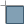Matrix de distancia

Measure distances between two point layers, and output results as a) Square distance matrix, b) Linear distance matrix, or c) Summary of distances. Can limit distances to the k nearest features.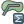Sum line length Calculate the total sum of line lengths for each polygon of a polygon vector layer.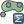Points in polygon Count the number of points that occur in each polygon of an input polygon vector layer.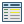List unique values List all unique values in an input vector layer field.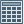Estadísticas básicas para campos de texto

Compute basic statistics (mean, std dev, N, sum, CV) on an input text field.Basic statistics for numeric field Compute basic statistics (mean, std dev, N, sum, CV) on an input numeric field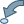Análisis del vecino más próximo

Compute nearest neighbor statistics to assess the level of clustering in a point vector layer.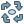Mean coordinate(s) Compute either the normal or weighted mean center of an entire vector layer, or multiple features based on a unique ID field.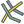Intersecciones de líneas

Locate intersections between lines, and output results as a point shapefile. Useful for locating road or stream intersections, ignores line intersections with length > 0.

Table tools 1: Default tools in Analysis group

## Herramientas de investigación¶

Icono

Herramienta

Propósito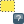Selección aleatoria

Randomly select n number of features, or n percentage of features.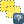Random selection within subsets Randomly select features within subsets based on a unique ID field.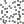Random points inside polygons Generate pseudo-random points over a polygon layer (variable number of point or fixed number of point).Puntos aleatorios en la extensión

Generate pseudo-random points over a given extent.Random points in layer bounds Generate pseudo-random points over bounds of a given input layer.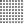Regular points Generate a regular grid of points over a specified region and export them as a point shapefile.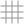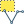Seleccionar por localización

Seleccionar entidades en función de su ubicación con respecto a otra capa para formar una nueva selección, o sumar o restar de la selección actual.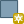Polígono a partir de extensión de la capa

Create a single rectangular polygon layer from the extent of an input raster or vector layer.

Table Tools 2: Default tools in Research group

## Herramientas de geoproceso¶

Icono

Herramienta

Propósito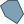Convex hull(s) Create minimum convex hull(s) for an input layer, or based on an ID field.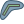Buffer with * fixed distance * distance field Create buffer(s) around features * based on fixed distance * based on distance field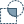Intersección

Overlay layers such that output contains areas where both layers intersect.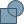Unión

Overlay layers such that output contains intersecting and non-intersecting areas.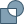Symmetrical difference Overlay layers such that output contains those areas of the input and difference layers that do not intersect.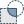Cortar

Overlay layers such that output contains areas that intersect the clip layer.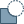Deferencia

Overlay layers such that output contains areas not intersecting the clip layer.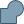Disolver

Merge features based on input field. All features with identical input values are combined to form one single feature.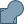Eliminate sliver polygons Merges selected features with the neighboring polygon with the largest area or largest common boundary.

Table Tools 3: Default tools in Geoprocessing group

## Geometry tools¶

Icono

Herramienta

Propósito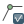Check geometry validity Check polygons for intersections, closed holes, and fix node ordering. You can choose the engine used by the in the options dialog, digitizing tab Change the Validate geometries value. There is two engines: QGIS and GEOS which have pretty different behavior. Another tools exists which shows different result as well: Topology Checker plugin and ‘must not have invalid geometries’ rule.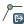Export/Add geometry columns Add vector layer geometry info to point (XCOORD, YCOORD), line (LENGTH), or polygon (AREA, PERIMETER) layer.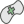Polygon centroids Calculate the true centroids for each polygon in an input polygon layer.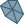Triangulación de Delaunay

Calculate and output (as polygons) the Delaunay triangulation of an input point vector layer.

Polígonos Voronoi

Calculate Voronoi polygons of an input point vector layer.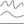Simplify geometry Generalize lines or polygons with a modified Douglas-Peucker algorithm.

Densificar geometrías

Densify lines or polygons by adding vertices.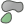Multipartes a partes sencillas

Convert multipart features to multiple singlepart features. Creates simple polygons and lines.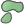Singleparts to multipart Merge multiple features to a single multipart feature based on a unique ID field.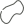Polygons to lines Convert polygons to lines, multipart polygons to multiple singlepart lines.Lines to polygons Convert lines to polygons, multipart lines to multiple singlepart polygons.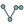Extract nodes Extract nodes from line and polygon layers and output them as points.

Table Tools 4: Default tools in Geometry group

Nota

The Simplify geometry tool can be used to remove duplicate nodes in line and polygon geometries. Just set the Simplify tolerance parameter to 0 and this will do the trick.

## Herramientas de gestión de datos¶

Icono

Herramienta

Propósito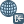Define current projection Specify the CRS for shapefiles whose CRS has not been defined.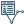Unir atributos por localización

Unir atributos adicionales a la capa de vectorial en función de su relación espacial. Los atributos de una capa vectorial se adjunta a la tabla de atributo de otra capa y se exporta como un archivo shape.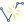Dividir capa vectorial

Split input layer into multiple separate layers based on input field.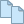Merge shapefiles to one Merge several shapefiles within a folder into a new shapefile based on the layer type (point, line, area).

Crear índice espacial

Create a spatial index for OGR- supported formats.

Table Tools 5: Default tools in Data management group